Test: Quadrilaterals- Assertion & Reason Type Questions

# Test: Quadrilaterals- Assertion & Reason Type Questions - Class 9

Test Description

## 10 Questions MCQ Test Additional Practice for Class 9 - Test: Quadrilaterals- Assertion & Reason Type Questions

Test: Quadrilaterals- Assertion & Reason Type Questions for Class 9 2023 is part of Additional Practice for Class 9 preparation. The Test: Quadrilaterals- Assertion & Reason Type Questions questions and answers have been prepared according to the Class 9 exam syllabus.The Test: Quadrilaterals- Assertion & Reason Type Questions MCQs are made for Class 9 2023 Exam. Find important definitions, questions, notes, meanings, examples, exercises, MCQs and online tests for Test: Quadrilaterals- Assertion & Reason Type Questions below.
 1 Crore+ students have signed up on EduRev. Have you?
Test: Quadrilaterals- Assertion & Reason Type Questions - Question 1

### Direction: In each of the following questions, a statement of Assertion is given followed by a corresponding statement of Reason just below it. Of the statements, mark the correct. Assertion : ABCD and PQRC are rectangles and Q is a midpoint of AC . Then DP = PC.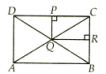Reason : The line segment joining the midpoint of any two sides of a triangle is parallel to the third side and equal to half of it.

Detailed Solution for Test: Quadrilaterals- Assertion & Reason Type Questions - Question 1

The correct option is B If both assertion and reason are true but reason is not the correct explanation of assertion
∴ P is the mid-point of DC
⇒ DP = PC [using converse of mid-point theorem]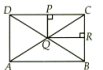∴ Assertion is true, reason is true but reason is not correct explanation of assertion.

Test: Quadrilaterals- Assertion & Reason Type Questions - Question 2

### Direction: In each of the following questions, a statement of Assertion is given followed by a corresponding statement of Reason just below it. Of the statements, mark the correct.Assertion : Two opposite angles of a parallelogram are (3x - 2º) and (50 - x)º . The measure of one of the angle is 37º.Reason : Opposite angles of a parallelogram are equal.

Detailed Solution for Test: Quadrilaterals- Assertion & Reason Type Questions - Question 2
Since, opposite angles of a parallelogram are equal.

Therefore, 3x - 2 = 50 - x

x = 13

One angle is 37º

Test: Quadrilaterals- Assertion & Reason Type Questions - Question 3

### Direction: In each of the following questions, a statement of Assertion is given followed by a corresponding statement of Reason just below it. Of the statements, mark the correct.Assertion : In ΔABC , E and F are the midpoints of AC and AB respectively. The altitude AP at BC intersects FE at Q. Then, AQ QP = .Reason : Q is the midpoint of AP.

Detailed Solution for Test: Quadrilaterals- Assertion & Reason Type Questions - Question 3
In ΔABC , E and F are midpoint of the sides AC

and AB respectively.

FE || BC [By mid-point theorem]

Now, in ΔABP, F is mid-point of AB and FQ || BP.

Q is mid-point of AP

AQ = QP.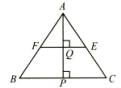Test: Quadrilaterals- Assertion & Reason Type Questions - Question 4

Direction: In each of the following questions, a statement of Assertion is given followed by a corresponding statement of Reason just below it. Of the statements, mark the correct.

Assertion : The angles of a quadrilateral are xº, (x - 10)º , (x + 30)º and (2x)º , the smallest angle is equal to 58º.

Reason : Sum of the angles of a quadrilateral is 360º.

Detailed Solution for Test: Quadrilaterals- Assertion & Reason Type Questions - Question 4
Given angles(in degrees) = x, x - 10, x = 30, 2x

sum of all angles 0 a quadrilateral is 360

=> x + x - 10 + x + 30 + 2x = 360

=> 5x = 20 = 360

=> 5x = 340

=> x = 68

smallest angle is x - 10

i.e. 68 - 10 = 58 degrees.

Test: Quadrilaterals- Assertion & Reason Type Questions - Question 5

Direction: In each of the following questions, a statement of Assertion is given followed by a corresponding statement of Reason just below it. Of the statements, mark the correct.

Assertion : A parallelogram consists of two congruent triangles.

Reason : Diagonal of a parallelogram divides it into two congruent triangles.

Detailed Solution for Test: Quadrilaterals- Assertion & Reason Type Questions - Question 5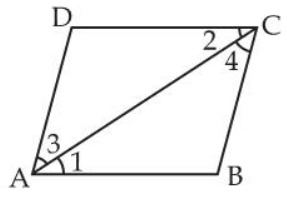In ΔBAC and ΔDCA

∠1 = ∠2

∠3 = ∠4 [alternate pair]

AC = AC (common)

ΔBAC ≅ ΔDCA (By ASA)

Therefore, diagonal of a parallelogram divides it into two congruent triangles.

Test: Quadrilaterals- Assertion & Reason Type Questions - Question 6

Direction: In each of the following questions, a statement of Assertion is given followed by a corresponding statement of Reason just below it. Of the statements, mark the correct.

Assertion : If the diagonals of a parallelogram ABCD are equal, then ∠ABC = 90º.

Reason : If the diagonals of a parallelogram are equal, it becomes a rectangle.

Detailed Solution for Test: Quadrilaterals- Assertion & Reason Type Questions - Question 6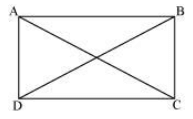Let ABCD be a parallelogram. To show that ABCD is a rectangle, we have to prove that one of its interior angles is 90º.

In ΔABC and ΔDCB,

AB = DC (Opposite sides of a parallelogram are equal)

BC = BC (Common)

AC = DB (Given)

∴ ΔABC ≅ ΔDCB (By SSS Congruence rule)

⇒ ∠ABC = ∠DCB

It is known that the sum of the measures of angles on the same side of transversal is 180º.

∠ABC + ∠DCB = 180º (AB || CD)

⇒ ∠ABC + ∠ABC = 180º

⇒ 2∠ABC = 180º

⇒ ∠ABC = 90º

Since ABCD is a parallelogram and one of its interior angles is 90º, ABCD is a rectangle.

Test: Quadrilaterals- Assertion & Reason Type Questions - Question 7

Direction: In each of the following questions, a statement of Assertion is given followed by a corresponding statement of Reason just below it. Of the statements, mark the correct.

Assertion : If the angles of a quadrilateral are in the ratio 2 : 3 : 7 : 6, then the measure of angles are 40º, 60º, 140º, 120º, respectively.

Reason : The sum of the angles of a quadrilateral is 360º.

Detailed Solution for Test: Quadrilaterals- Assertion & Reason Type Questions - Question 7
2x + 3x + 7x + 6x = 360º

=> 18x = 360º

=> x = 360º/18 = 20º

First angle = 2 × 20º = 40º

Second angle = 3 × 20º = 60º

Third angle = 7 x 20º = 140º

Fourth angle = 6 x 20º = 120º

Test: Quadrilaterals- Assertion & Reason Type Questions - Question 8

Direction: In each of the following questions, a statement of Assertion is given followed by a corresponding statement of Reason just below it. Of the statements, mark the correct.

Assertion : ABCD is a square. AC and BD intersect at O. The measure of ∠AOB = 90º.

Reason : Diagonals of a square bisect each other at right angles.

Detailed Solution for Test: Quadrilaterals- Assertion & Reason Type Questions - Question 8
Since, diagonals of a square bisect each other at right angles.

∠AOB = 90º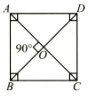Test: Quadrilaterals- Assertion & Reason Type Questions - Question 9

Direction: In each of the following questions, a statement of Assertion is given followed by a corresponding statement of Reason just below it. Of the statements, mark the correct.

Assertion : In ΔABC , median AD is produced to X such that AD DX = . Then ABXC is a parallelogram.

Reason : Diagonals AX and BC bisect each other at right angles.

Detailed Solution for Test: Quadrilaterals- Assertion & Reason Type Questions - Question 9
In quadrilateral ABXC , we have

BD = DC [Given]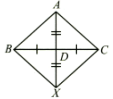So, diagonals AX and BC bisect each other but not

at right angles.

Therefore, ABXC is a parallelogram

Test: Quadrilaterals- Assertion & Reason Type Questions - Question 10

Direction: In each of the following questions, a statement of Assertion is given followed by a corresponding statement of Reason just below it. Of the statements, mark the correct.

Assertion : The consecutive sides of a quadrilateral have one common point.

Reason : The opposite sides of a quadrilateral have two common point.

Detailed Solution for Test: Quadrilaterals- Assertion & Reason Type Questions - Question 10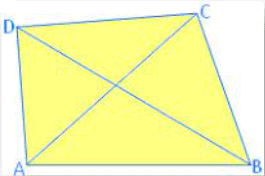Two sides of a quadrilateral are called its opposite sides if they do not have a common end point.

In the given figure, (AB, DC) and (AD, BC) are two pairs of opposite sides of quadrilateral ABCD.

Two angles of a quadrilateral having a common arm are called its adjacent angles.

## Additional Practice for Class 9

266 docs|65 tests